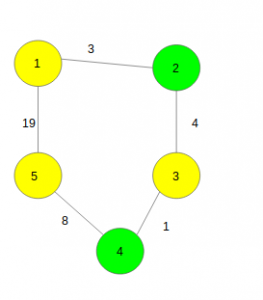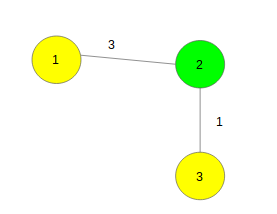# Find the shortest distance between any pair of two different good nodes

Given a weighted undirected connected graph with N nodes and M edges. Some of the nodes are marked as good. The task is to find the shortest distance between any pair of two different good nodes.
Note: Nodes marked as yellow in the below examples are considered to be good nodes.

Examples:

`Input :````Output : 7
Explanation :
Pairs of Good Nodes and distance between them are:
(1 to 3) -> distance: 7,
(3 to 5) -> distance: 9,
(1 to 5) -> distance: 16,
out of which 7 is the minimum.

Input :````Output : 4`

Approach: Let us start by thinking of an algorithm to solve a simpler version of the given problem wherein all edges are of weight 1.

• Pick a random good node and perform a BFS from this point and stop at the first level saywhich contains another good node.
• We know that the minimum distance between any two good nodes can’t be more than s. So we again take a good node at random which is not already taken before and perform a BFS again. If we don’t find any special node in s distance, we terminate the search. If we do, then we update the value of s and repeat the procedure with some other special node taken at random.

We can apply a similar algorithm when weights are multiple.

Below is the implementation of the above approach:

## C++

 `// C++ program to find the shortest pairwise` `// distance between any two different good nodes.` `#include ` `using` `namespace` `std;`   `#define N 100005` `const` `int` `MAXI = 99999999;`   `// Function to add edges` `void` `add_edge(vector > gr[], ``int` `x,` `              ``int` `y, ``int` `weight)` `{` `    ``gr[x].push_back({ y, weight });` `    ``gr[y].push_back({ x, weight });` `}`   `// Function to find the shortest` `// distance between any pair of` `// two different good nodes` `int` `minDistance(vector > gr[], ``int` `n,` `                ``int` `dist[], ``int` `vis[], ``int` `a[], ``int` `k)` `{` `    ``// Keeps minimum element on top` `    ``priority_queue, vector >,` `                   ``greater > > q;`   `    ``// To keep required answer` `    ``int` `ans = MAXI;`   `    ``for` `(``int` `i = 1; i <= n; i++) {` `        ``// If it is not good vertex` `        ``if` `(!a[i])` `            ``continue``;`   `        ``// Keep all vertices not visited` `        ``// and distance as MAXI` `        ``for` `(``int` `j = 1; j <= n; j++) {` `            ``dist[j] = MAXI;` `            ``vis[j] = 0;` `        ``}`   `        ``// Distance from ith vertex to ith is zero` `        ``dist[i] = 0;`   `        ``// Make queue empty` `        ``while` `(!q.empty())` `            ``q.pop();`   `        ``// Push the ith vertex` `        ``q.push({ 0, i });`   `        ``// Count the good vertices` `        ``int` `good = 0;`   `        ``while` `(!q.empty()) {` `            ``// Take the top element` `            ``int` `v = q.top().second;`   `            ``// Remove it` `            ``q.pop();`   `            ``// If it is already visited` `            ``if` `(vis[v])` `                ``continue``;` `            ``vis[v] = 1;`   `            ``// Count good vertices` `            ``good += a[v];`   `            ``// If distance from vth vertex` `            ``// is greater than ans` `            ``if` `(dist[v] > ans)` `                ``break``;`   `            ``// If two good vertices are found` `            ``if` `(good == 2 and a[v]) {` `                ``ans = min(ans, dist[v]);` `                ``break``;` `            ``}`   `            ``// Go to all adjacent vertices` `            ``for` `(``int` `j = 0; j < gr[v].size(); j++) {` `                ``int` `to = gr[v][j].first;` `                ``int` `weight = gr[v][j].second;`   `                ``// if distance is less` `                ``if` `(dist[v] + weight < dist[to]) {` `                    ``dist[to] = dist[v] + weight;` `                    ``q.push({ dist[to], to });` `                ``}` `            ``}` `        ``}` `    ``}`   `    ``// Return the required answer` `    ``return` `ans;` `}`   `// Driver code` `int` `main()` `{` `    ``// Number of vertices and edges` `    ``int` `n = 5, m = 5;`   `    ``vector > gr[N];`   `    ``// Function call to add edges` `    ``add_edge(gr, 1, 2, 3);` `    ``add_edge(gr, 1, 2, 3);` `    ``add_edge(gr, 2, 3, 4);` `    ``add_edge(gr, 3, 4, 1);` `    ``add_edge(gr, 4, 5, 8);`   `    ``// Number of good nodes` `    ``int` `k = 3;`   `    ``int` `a[N], vis[N], dist[N];`   `    ``// To keep good vertices` `    ``a = a = a = 1;`   `    ``cout << minDistance(gr, n, dist, vis, a, k);`   `    ``return` `0;` `}`

## Java

 `// Java program to find the shortest pairwise` `// distance between any two different good nodes.` `import` `java.util.ArrayList;` `import` `java.util.Comparator;` `import` `java.util.PriorityQueue;`   `class` `GFG{`   `static` `class` `Pair ` `{` `    ``int` `first, second;`   `    ``public` `Pair(``int` `first, ``int` `second) ` `    ``{` `        ``this``.first = first;` `        ``this``.second = second;` `    ``}`   `    ``public` `Pair()` `    ``{}` `}`   `static` `final` `int` `N = ``100005``;` `static` `final` `int` `MAXI = ``99999999``;`   `// Function to add edges` `static` `void` `add_edge(ArrayList gr[], ` `                     ``int` `x, ``int` `y, ``int` `weight)` `{` `    ``gr[x].add(``new` `Pair(y, weight));` `    ``gr[y].add(``new` `Pair(x, weight));` `}`   `// Function to find the shortest` `// distance between any pair of` `// two different good nodes` `static` `int` `minDistance(ArrayList gr[], ``int` `n, ` `                       ``int` `dist[], ``int` `vis[],` `                       ``int` `a[], ``int` `k)` `{` `    `  `    ``// Keeps minimum element on top` `    ``PriorityQueue q = ``new` `PriorityQueue<>(` `        ``new` `Comparator()` `    ``{` `        ``public` `int` `compare(Pair p1, Pair p2)` `        ``{` `            ``if` `(p1.first == p2.first)` `            ``{` `                ``return` `p1.second - p2.second;` `            ``}` `            ``return` `p1.first - p2.first;` `        ``}` `    ``});`   `    ``// To keep required answer` `    ``int` `ans = MAXI;`   `    ``for``(``int` `i = ``1``; i <= n; i++)` `    ``{` `        `  `        ``// If it is not good vertex` `        ``if` `(a[i] == ``0``)` `            ``continue``;`   `        ``// Keep all vertices not visited` `        ``// and distance as MAXI` `        ``for``(``int` `j = ``1``; j <= n; j++) ` `        ``{` `            ``dist[j] = MAXI;` `            ``vis[j] = ``0``;` `        ``}`   `        ``// Distance from ith vertex ` `        ``// to ith is zero` `        ``dist[i] = ``0``;`   `        ``// Make queue empty` `        ``while` `(!q.isEmpty())` `            ``q.poll();`   `        ``// Push the ith vertex` `        ``q.add(``new` `Pair(``0``, i));`   `        ``// Count the good vertices` `        ``int` `good = ``0``;`   `        ``while` `(!q.isEmpty())` `        ``{` `            `  `            ``// Take the top element` `            ``int` `v = q.peek().second;`   `            ``// Remove it` `            ``q.poll();`   `            ``// If it is already visited` `            ``if` `(vis[v] != ``0``)` `                ``continue``;` `            ``vis[v] = ``1``;`   `            ``// Count good vertices` `            ``good += a[v];`   `            ``// If distance from vth vertex` `            ``// is greater than ans` `            ``if` `(dist[v] > ans)` `                ``break``;`   `            ``// If two good vertices are found` `            ``if` `(good == ``2` `&& a[v] != ``0``) ` `            ``{` `                ``ans = Math.min(ans, dist[v]);` `                ``break``;` `            ``}`   `            ``// Go to all adjacent vertices` `            ``for``(``int` `j = ``0``; j < gr[v].size(); j++) ` `            ``{` `                ``int` `to = gr[v].get(j).first;` `                ``int` `weight = gr[v].get(j).second;`   `                ``// If distance is less` `                ``if` `(dist[v] + weight < dist[to]) ` `                ``{` `                    ``dist[to] = dist[v] + weight;` `                    ``q.add(``new` `Pair(dist[to], to));` `                ``}` `            ``}` `        ``}` `    ``}`   `    ``// Return the required answer` `    ``return` `ans;` `}`   `// Driver code` `public` `static` `void` `main(String[] args)` `{` `    `  `    ``// Number of vertices and edges` `    ``int` `n = ``5``, m = ``5``;`   `    ``@SuppressWarnings``(``"unchecked"``)` `    ``ArrayList[] gr = ``new` `ArrayList[N];`   `    ``for``(``int` `i = ``0``; i < N; i++) ` `    ``{` `        ``gr[i] = ``new` `ArrayList();` `    ``}`   `    ``// Function call to add edges` `    ``add_edge(gr, ``1``, ``2``, ``3``);` `    ``add_edge(gr, ``1``, ``2``, ``3``);` `    ``add_edge(gr, ``2``, ``3``, ``4``);` `    ``add_edge(gr, ``3``, ``4``, ``1``);` `    ``add_edge(gr, ``4``, ``5``, ``8``);`   `    ``// Number of good nodes` `    ``int` `k = ``3``;`   `    ``int``[] a = ``new` `int``[N], ` `        ``vis = ``new` `int``[N], ` `       ``dist = ``new` `int``[N];`   `    ``// To keep good vertices` `    ``a[``1``] = a[``3``] = a[``5``] = ``1``;`   `    ``System.out.println(minDistance(` `        ``gr, n, dist, vis, a, k));` `}` `}`   `// This code is contributed by sanjeev2552`

Output:

`7`My Personal Notes arrow_drop_uppawanasipugmailcom

If you like GeeksforGeeks and would like to contribute, you can also write an article using contribute.geeksforgeeks.org or mail your article to contribute@geeksforgeeks.org. See your article appearing on the GeeksforGeeks main page and help other Geeks.

Please Improve this article if you find anything incorrect by clicking on the "Improve Article" button below.